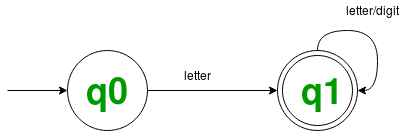# DFA (Recognizer) for valid Pascal identifiers

Problem – Implement a recognizer for pascal identifiers based on a DFA that accepts strings belonging to the definition of the language of the same.

Here is a regular definition for the set of Pascal identifiers that are defined as the set of strings of letters and digits beginning with a letter.

```letter : A | B | . . . | Z | a | b | . . . | z
digit  : 0 | 1 | 2 | . . . | 9
ID : letter (letter | digit)* ```

The regular expression ID is the pattern for the Pascal identifier token and defines letter and digit where a letter is a regular expression for the set of all upper-case and lowercase letters in the alphabet and digit is regular for the set of all decimal digits.

State diagram of the DFAWorking code for the recognizer:

 `// C++ program to implement DFA based regonizer that accepts ` `// all strings which follow the language ` `// L = { letter (letter | digit)* } ` ` `  `#include ` `#include ` `using` `namespace` `std; ` ` `  `// dfa tells the number associated ` `// with the present state ` `int` `dfa; ` ` `  `// This function is for ` `// the starting state (zeroth) of DFA ` `void` `start(``char` `c) ` `{ ` `    ``if` `(``isalpha``(c)) ` `        ``dfa = 1; ` `    ``else` ` `  `// -1 is used to check for any invalid symbol ` `        ``dfa = -1;  ` `} ` ` `  `// This function is for the first state of DFA ` `void` `state1(``char` `c) ` `{ ` `    ``if` `(``isalnum``(c)) ` `        ``dfa = 1; ` `    ``else` `        ``dfa = -1; ` `} ` ` `  `bool` `DFA_for_ID(string token) ` `{ ` `    ``dfa = 0; ` `    ``int` `i, len = token.length(); ` `    ``for` `(i = 0; i < len; i++) { ` `        ``if` `(dfa == 0) ` `            ``start(token[i]); ` `        ``else` `if` `(dfa == 1) ` `            ``state1(token[i]); ` `        ``else` `            ``return` `0; ` `    ``} ` `    ``if` `(dfa == 1) ` `        ``return` `1; ` `    ``else` `        ``return` `0; ` `} ` ` `  `// driver code ` `int` `main() ` `{ ` `    ``string input = ``"Geeks for Geeks is 9ice platfo\$m for every1  "``; ` ` `  `// to seprate all the tokens by space in the string  ` `// and cheking for each token ` `    ``stringstream ss(input);  ` `    ``string token; ` `    ``while` `(ss >> token) { ` `        ``bool` `isValid = DFA_for_ID(token); ` `        ``if` `(isValid) ` `            ``cout << token << ``" : "` `                 ``<< ``"Valid"` `<< endl; ` `        ``else` `            ``cout << token << ``" : "` `                 ``<< ``"Invalid"` `<< endl; ` `    ``} ` `    ``return` `0; ` `} `

Output:

```Geeks : Valid
for : Valid
Geeks : Valid
is : Valid
9ice : Invalid
platfo\$m : Invalid
for : Valid
every1 : Valid```

GeeksforGeeks has prepared a complete interview preparation course with premium videos, theory, practice problems, TA support and many more features. Please refer Placement 100 for details

My Personal Notes arrow_drop_upCheck out this Author's contributed articles.

If you like GeeksforGeeks and would like to contribute, you can also write an article using contribute.geeksforgeeks.org or mail your article to contribute@geeksforgeeks.org. See your article appearing on the GeeksforGeeks main page and help other Geeks.

Please Improve this article if you find anything incorrect by clicking on the "Improve Article" button below.

Article Tags :

Be the First to upvote.

Please write to us at contribute@geeksforgeeks.org to report any issue with the above content.